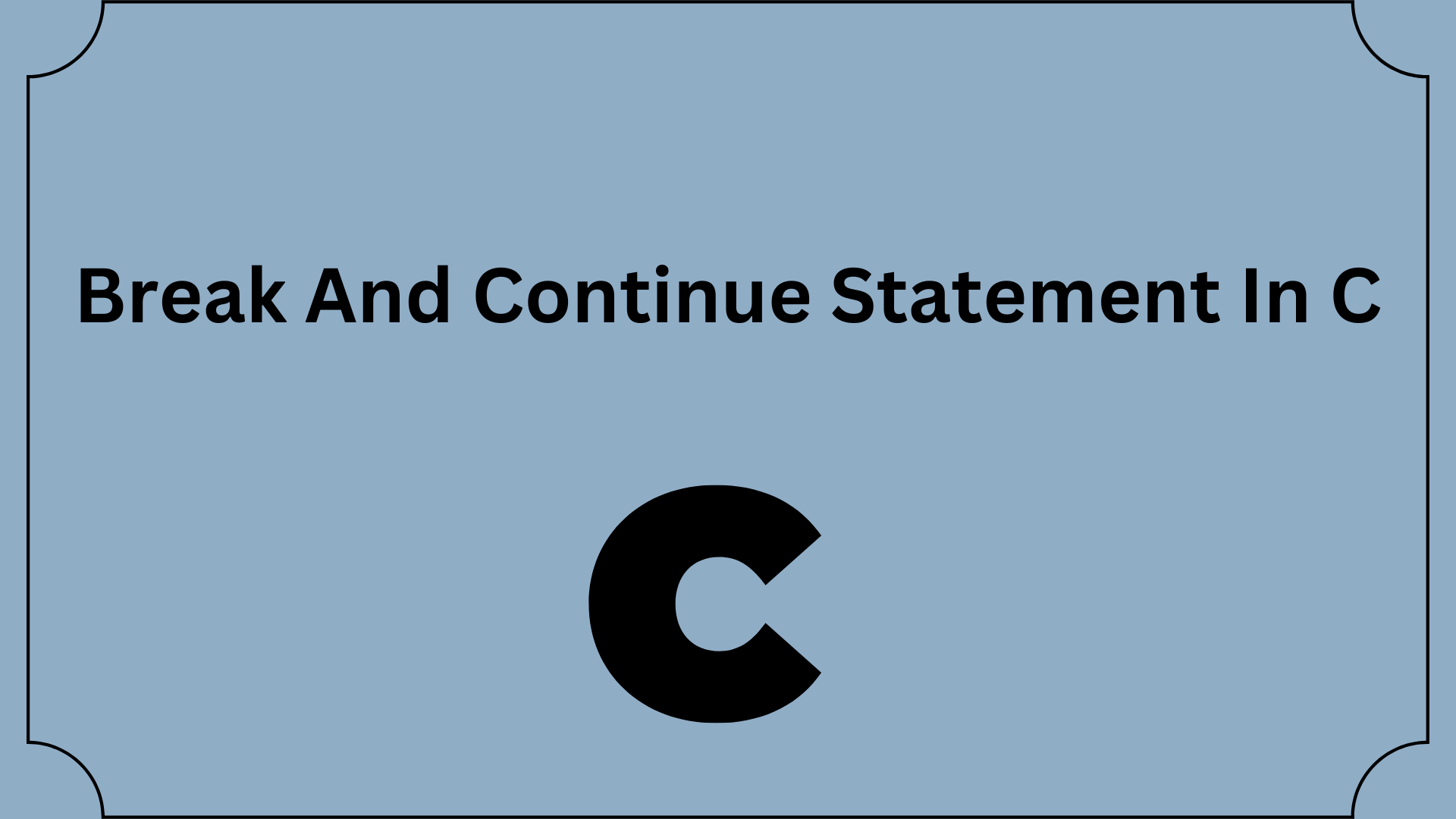## Break And Continue Statement In C

In earlier tutorials, we learned about loops. With the aid of examples, we will learn how to use a break and continue statement in this tutorial.

The distinction between the C break and continue statements will be covered in this article.

Although they are the same kind of statements that are used to change a program’s flow, they differ in a few ways.

Using the break statement, the smallest enclosing loop is ended (i.e., while, do-while, for loop, or switch statement). The software to demonstrate this is below:

Contents

## C break statement (break and continue statement)

Regardless of the conditions given for the loop, we have a mechanism to immediately exit any of the C loops at any time.

Using the break keyword accomplishes this.

To put it simply, the break statement is a loop control statement that ends a loop instantly.

Break statements are generally used when we are unsure of the precise number of loop iterations to perform or when we wish to end the loop based on a condition.

When the break statement is encountered, the loop is immediately terminated. The syntax is:

`break;`

In the loop, the break statement is nearly typically combined with an if…else expression.

## Example 1: break statement(break and continue statement)

```// Program to calculate the sum of numbers (10 numbers max)
// If the user enters a negative number, the loop terminates

#include <stdio.h>

int main() {
int i;
double number, sum = 0.0;

for (i = 1; i <= 10; ++i) {
printf("Enter n%d: ", i);
scanf("%lf", &number);

// if the user enters a negative number, break the loop
if (number < 0.0) {
break;
}

sum += number; // sum = sum + number;
}

printf("Sum = %.2lf", sum);

return 0;
}```

## Output

Enter n1: 2.4
Enter n2: 4.5
Enter n3: 3.4
Enter n4: -3
Sum = 10.30

This application can add up to ten different numbers. Why only 10 numbers in total? It’s because the break statement is executed if the user provides a negative value. The for loop will come to a close, and the sum will now be visible.

The break can also be combined with the switch statement in C. It will be covered in the next tutorial.

## C continue statement (break and continue statement)

further statement: This sentence skips the remaining loop statement and initiates the loop’s subsequent iteration. The software to demonstrate this is below:

One of the simplest and most fundamental keywords in C/C++ that offers programmers control over loops is this one.

The continuation is exactly as the name implies. Since we utilize this in loops, the remaining body of the current loop will be skipped, and the next iteration will begin.

This can be used within any loop, including the for, while, and do-while loops.

In plain English, 1. A for/while loop’s final segment is skipped using the continue command.

We proceed to the loop condition check in the subsequent iteration.

Programmers can use this to be versatile in how they approach control loops.

The loop’s current iteration is skipped by the continue statement in favor of the following one. The syntax is:

`continue;`

With the if…else statement, the continue statement is usually always utilized.

## Example 2: continue statement(break and continue statement)

```// Program to calculate the sum of numbers (10 numbers max)
// If the user enters a negative number, it's not added to the result

#include <stdio.h>
int main() {
int i;
double number, sum = 0.0;

for (i = 1; i <= 10; ++i) {
printf("Enter a n%d: ", i);
scanf("%lf", &number);

if (number < 0.0) {
continue;
}

sum += number; // sum = sum + number;
}

printf("Sum = %.2lf", sum);

return 0;
}```

## Output

Enter n1: 1.1
Enter n2: 2.2
Enter n3: 5.5
Enter n4: 4.4
Enter n5: -3.4
Enter n6: -45.5
Enter n7: 34.5
Enter n8: -4.2
Enter n9: -1000
Enter n10: 12
Sum = 59.70

When a user inputs a positive number into this program, the sum is calculated using the sum += number; command.

The continue statement is run and skips the negative number from the calculation when the user submits a negative number.

You Can Try The Online Compiler:

C Compiler